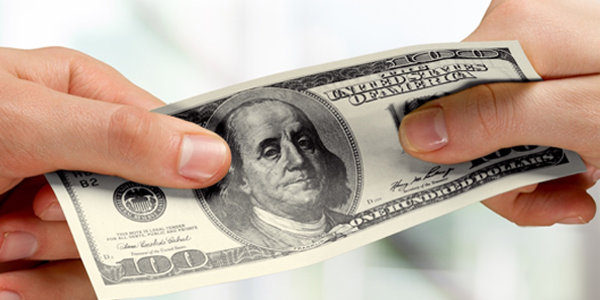Home / Money Blog

## How Much am I Really Paying?Note: You can use any financial calculator to do this problem, but if you want the BEST, you can get our 10bii Financial Calculator for iOS, Android, Mac, and Windows!THE SCENARIO Last time, I discussed buying a house with a hard money loan (or 'buying it with hard money'). To summarize, the loan would be for \$60,000 and it would last for 8 months, with an interest rate of 11% and 2 points. At the end, I'd pay the principal, interest, and points, in an amount totaling \$65,743.78. The question: The note's interest rate is 11%, but the points change my effective borrowing rate. What's my effective annual interest rate on the \$60,000 I'm borrowing?
THE SOLUTION This one is pretty straightforward. First things first, make sure the calculator is using 12 Payments per Year. N: 8 (I pay back the loan after 8 months) I/YR: (This is what I'm trying to find) PV: 60,000 (I borrow \$60,000 in the beginning) PMT: 0 (During the course of the loan, no payments are due) FV: \$65,743.78 (At the end, I repay \$65,743.78)

I'm paying an effective annual rate of 13.79% due to the combination of interest rate, points, and the short time frame after which I repay the loan.

What do you think? Would you borrow at a nearly 14% effective rate? Why or why not? Let us know in the comments!